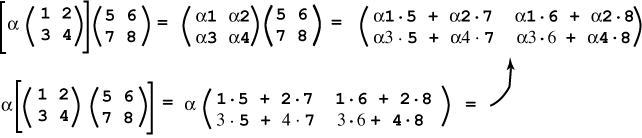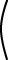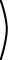YES    c(AB)  =  (cA)B for scalar c.

# Scalar Factor

Recall that cA means that every element of A is multiplied by c. Here is a demonstration of the rule:Often a matrix-matrix multiplication can be simpified by factoring one of the matrices. See the question.

### QUESTION 10:

Compute the following:``` 10 0 20 20 40 10 0 20 10 `````` -2 2 1 1 2 -2 ```